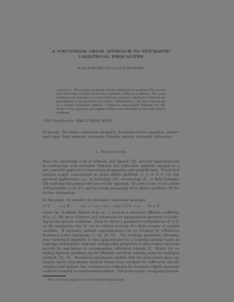Repository: Freie Universität Berlin, Math Department

# A polynomial chaos approach to stochastic variational inequalities

Forster, R. and Kornhuber, R. (2010) A polynomial chaos approach to stochastic variational inequalities. Journal of Numerical Mathematics, 18 (4). pp. 235-255. ISSN 1570-2820Preview

350kB

Official URL: http://dx.doi.org/10.1515/jnum.2010.012

## Abstract

We consider stochastic elliptic variational inequalities of the second kind involving a bilinear form with stochastic diffusion coefficient. We prove existence and uniqueness of weak solutions, propose a stochastic Galerkin approximation of an equivalent parametric reformulation, and show equivalence to a related collocation method. Numerical experiments illustrate the efficiency of our approach and suggest similar error estimates as for linear elliptic problems.

Item Type: Article Stochastic variational inequality, Karhunen–Loève expansion, polynomial chaos, finite elements, stochastic Galerkin method, stochastic collocation Mathematical and Computer Sciences > Mathematics > Numerical Analysis Department of Mathematics and Computer Science > Institute of Mathematics 1801 Ekaterina Engel 18 Feb 2016 10:01 03 Mar 2017 14:41

Repository Staff Only: item control page